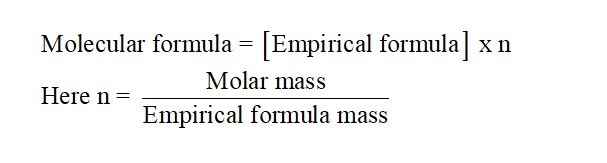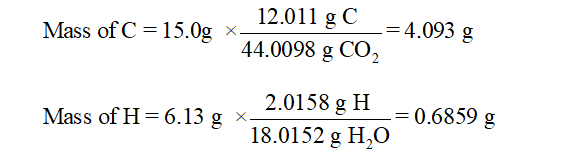# An unknown compound contains only C , H , and O . Combustion of 7.50 g of this compound produced 15.0 g CO2 and 6.13 gH2O . What is the empirical formula of the unknown compound? Insert subscripts as needed.

Question
8 views

An unknown compound contains only C , H , and O . Combustion of 7.50 g of this compound produced 15.0 g CO2 and 6.13 gH2O . What is the empirical formula of the unknown compound? Insert subscripts as needed.

check_circle

Step 1

The empirical formula is the chemical formula of any compound that represents the proportions of each elements that is present in the given compound. It does not show the actual number of element as well as cannot give any information about arrangement of atoms in the formula of compound.

Step 2

The relation between molecular formula and empirical formula is:Step 3

To get the chemical formula or empirical formula;

Mass of compound = 7.50 g

Mass of CO2 = 15.0 g

Mass of H2O = 6.13 g

Convert grams of C and H to their respective amount of moles....

### Want to see the full answer?

See Solution

#### Want to see this answer and more?

Solutions are written by subject experts who are available 24/7. Questions are typically answered within 1 hour.*

See Solution
*Response times may vary by subject and question.
Tagged in

### Chemistry